Courses

# Test: Simplification Of A Force And Couple System

## 30 Questions MCQ Test Engineering Mechanics - Notes, Videos, MCQs & PPTs | Test: Simplification Of A Force And Couple System

Description
This mock test of Test: Simplification Of A Force And Couple System for Mechanical Engineering helps you for every Mechanical Engineering entrance exam. This contains 30 Multiple Choice Questions for Mechanical Engineering Test: Simplification Of A Force And Couple System (mcq) to study with solutions a complete question bank. The solved questions answers in this Test: Simplification Of A Force And Couple System quiz give you a good mix of easy questions and tough questions. Mechanical Engineering students definitely take this Test: Simplification Of A Force And Couple System exercise for a better result in the exam. You can find other Test: Simplification Of A Force And Couple System extra questions, long questions & short questions for Mechanical Engineering on EduRev as well by searching above.
QUESTION: 1

### The basic type of motion of a body is translation motion only.

Solution:

The basic types of the motion of the body are translation and rotational. In which translation means the motion is a straight motion while the in the rotational motion the body is moving of the body along the axis.

QUESTION: 2

### Find the total resultant force acting horizontally in the given figure below, consisting of a beam with various sections?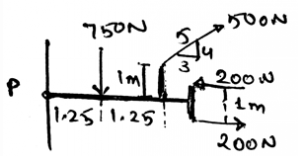Solution:

The couple is simplified by taking the direction of the rotation of the body as positive or negative. That is if the clockwise direction is positive then the anti-clockwise direction is taken as negative. If the couple is a vector it can be easily simplified by taking the components.

QUESTION: 3

### Principle of transmissibility is

Solution:

Principle of transmissibility states that the force acting on the body is a sliding vector. That is it can be applied at any point of the body. It will give the same effect as if applied at any point other that the specific point. Thus the answer.

QUESTION: 4

Coplanar forces are not easily simplified in the simplification of the force and couple system.

Solution:

The coplanar forces can be easily simplified in the simplification of the force and couple system. This is not only for the coplanar system of the forces, but for any of the dimension vector. Which means the answer is false.

QUESTION: 5

What will be the total resultant force acting vertically in the figure given below consisting of a beam with various sections?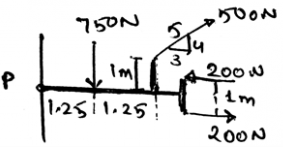Solution:

The couple is simplified by taking the direction of the rotation of the body as positive or negative. That is if the clockwise direction is positive then the anti-clockwise direction is taken as negative. If the couple is a vector it can be easily simplified by taking the components.

QUESTION: 6

The simplification of the couple is done on the basis of the ______________

Solution:

The couple is simplified by taking the direction of the rotation of the body as positive or negative. That is if the clockwise direction is positive then the anti-clockwise direction is taken as negative. If the couple is a vector it can be easily simplified by taking the components.

QUESTION: 7

The simplification of the forces on the axis is done as______________

Solution:

A particular system of the rules is followed that is if the upward direction is taken as positive then the downward direction is taken as negative. This is same as done with the couple in the 2D. That is the forces can be easily simplified. If taken in the vector form then the task is even easier.

QUESTION: 8

Find the total resultant force acting horizontally in the given figure consisting of a beam of ‘L’ shape?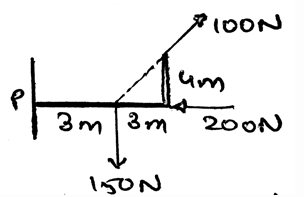Solution:

The couple is simplified by taking the direction of the rotation of the body as positive or negative. That is if the clockwise direction is positive then the anti-clockwise direction is taken as negative. If the couple is a vector it can be easily simplified by taking the components.

QUESTION: 9

The couple is a scalar quantity and the force is vector quantity and hence only force can be simplified as __________

Solution:

Both of them are vector quantities. And both of them can be easily simplified. If taken in the vector form then the task is even easier. Thus it is not necessary for the force or the couple to be vector only, even if the magnitude is taken, the simplification is done in the 2D.

QUESTION: 10

The force and the couple can’t be simplified together as one is the cause and the other is the effect.

Solution:

Both of them are vector quantities. And both of them can be easily simplified. If taken in the vector form then the task is even easier. Thus it is not necessary for the force or the couple to be vector only, even if the magnitude is taken, the simplification is done in the 2D.

QUESTION: 11

The main moto of the simplification is to ______________

Solution:

The main moto of the simplification is to reduce the calculation. The forces are simplified and the couples too are simplified. This gives us the total force and the total moment acting on the body. This gives us a single value of the respective thing and hence reduces the labour.

QUESTION: 12

Find the total resultant force acting vertically in the given figure below consisting of a beam of ‘L’ shape.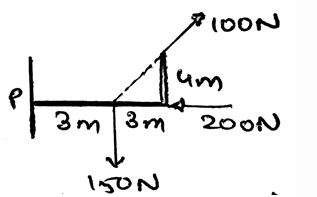Solution:

The couple is simplified by taking the direction of the rotation of the body as positive or negative. That is if the clockwise direction is positive then the anti-clockwise direction is taken as negative. If the couple is a vector it can be easily simplified by taking the components.

QUESTION: 13

The couple is simplified easily by the help of right hand rule. But the forces simplification is not possible as there is no such system of rules so that the forces can be simplified.

Solution:

Both of them are vector quantities. And both of them can be easily simplified. If taken in the vector form then the task is even easier. Thus it is not necessary for the force or the couple to be vector only, even if the magnitude is taken, the simplification is done in the 2D. Forces are simplified on the basis of the algebra.

QUESTION: 14

The couple moment and the force is divided to get the distance of the axis from the point of action of the force.

Solution:

The couple is the cross product of the force and the perpendicular distance between the forces. And if the division of the both is done than the resulting quantity is the distance only. But care must be taken that the product is the cross product and hence sine angle must be divided too to get the distance.

QUESTION: 15

Find the total resultant moment acting vertically in the given figure below consisting of a beam of ‘L’ shape.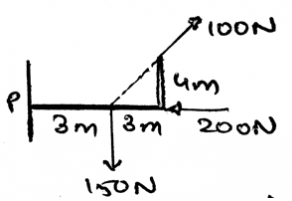Solution:

The couple is simplified by taking the direction of the rotation of the body as positive or negative. That is if the clockwise direction is positive then the anti-clockwise direction is taken as negative. If the couple is a vector it can be easily simplified by taking the components.

QUESTION: 16

What is a collinear system of forces?

Solution:

The force system having all the forces emerging from a point is called the collinear system of force. This is a type of system of the force, which is easy in the simplification. This is because as the forces are the vector quantity, the vector math is applied and the simplification is done.

QUESTION: 17

Find the total resultant force acting in the given figure consisting of a beam with various sections.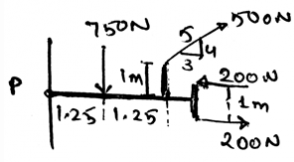Solution:

The couple is simplified by taking the direction of the rotation of the body as positive or negative. That is if the clockwise direction is positive then the anti-clockwise direction is taken as negative. After this the forces are a determined and are added vetorially to get the resultant force.

QUESTION: 18

In the collinear force system a net force is being made after all the simplification done on all the forces.

Solution:

The answer is obvious yes. As the simplification means addition and the subtraction of the forces. This results in the simplification of the forces and thus gives us a single force. It is in a particular direction and hence the statement is true.

QUESTION: 19

Just like the collinear forces there are collinear couple and the net moment is taken out from it.

Solution:

Both of them are vector quantities. And both of them can be easily simplified. If taken in the vector form then the task is even easier. Thus it is not necessary for the force or the couple to be vector only, even if the magnitude is taken, the simplification is done in the 2D.

QUESTION: 20

Just like the collinear force system there is a system of the parallel forces.

Solution:

There is a system so called the parallel system of the forces. This is another type of the forces. The simplification of this type of the forces is also very easy. This means that the forces in a certain direction is taken as positive and in the other direction is negative and hence the simplification is done.

QUESTION: 21

Find the total resultant moment acting in the given figure consisting of a beam with various sections.

Solution:

The couple is simplified by taking the direction of the rotation of the body as positive or negative. That is if the clockwise direction is positive then the anti-clockwise direction is taken as negative. If the couple is a vector it can be easily simplified by taking the components.

QUESTION: 22

The system of the collinear and the parallel force are simplified as ______________

Solution:

The simplification is easy. The simplified force system gives us a net force and the parallel force system gives us a simplified force, and then we add it vectorially. Thus the vector addition at the last can be easily done and thus both can be simplified.

QUESTION: 23

The couple and the other two force systems can be easily simplified.

Solution:

Both of them are vector quantities. And both of them can be easily simplified. If taken in the vector form then the task is even easier. Thus it is not necessary for the force or the couple to be vector only, even if the magnitude is taken, the simplification is done in the 2D.

QUESTION: 24

The resultant force is equal to the _______ of all the forces.

Solution:

As the simplification means addition and the subtraction of the forces. This results in the simplification of the forces and thus gives us a single force. It is in a particular direction. But if one is considering the direction with the magnitude of the force, we can say that the resultant is the sum of all the forces.

QUESTION: 25

The couple is simplified easily by the help of left hand rule. But the forces simplification is not possible as there is no such system of rules so that the forces can be simplified.

Solution:

Both of them are vector quantities. And both of them can be easily simplified. If taken in the vector form then the task is even easier. Thus it is not necessary for the force or the couple to be vector only, even if the magnitude is taken, the simplification is done in the 2D. Forces are simplified on the basis of the algebra.

QUESTION: 26

The forces of the parallel system and the couple can’t be simplified together as one is the cause and the other is the effect.

Solution:

Both of them are vector quantities. And both of them can be easily simplified. If taken in the vector form then the task is even easier. Thus it is not necessary for the force or the couple to be vector only, even if the magnitude is taken, the simplification is done in the 2D.

QUESTION: 27

The forces of the collinear system and the couple can’t be simplified together as one is the cause and the other is the effect.

Solution:

Both of them are vector quantities. And both of them can be easily simplified. If taken in the vector form then the task is even easier. Thus it is not necessary for the force or the couple to be vector only, even if the magnitude is taken, the simplification is done in the 2D.

QUESTION: 28

Find the angle between the horizontal and vertical forces acting in the given figure consisting of a beam with various sections.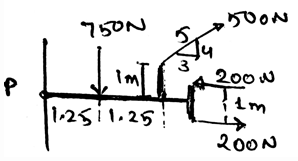Solution:

The couple is simplified by taking the direction of the rotation of the body as positive or negative. As soon as the horizontal and the vertical forces are determined we can get the angle between them. This can be done by using the tangent trigonometry function.

QUESTION: 29

Find the total resultant moment acting in the given figure consisting of a beam with various sections.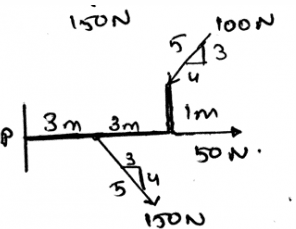Solution:

The couple is simplified by taking the direction of the rotation of the body as positive or negative. As soon as the horizontal and the vertical forces are determined we can get the angle between them. This can be done by using the tangent trigonometry function.

QUESTION: 30

Find the total resultant force acting in the given figure consisting of a beam with various sections.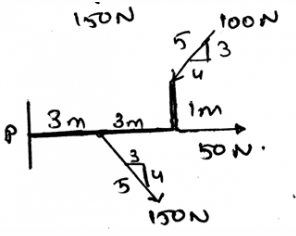Solution:

The couple is simplified by taking the direction of the rotation of the body as positive or negative. That is if the clockwise direction is positive then the anti-clockwise direction is taken as negative. After this the forces are a determined and are added vetorially to get the resultant force.